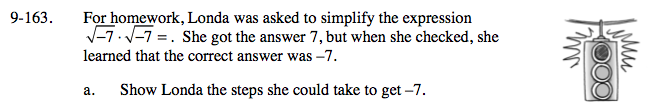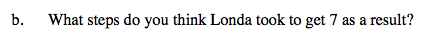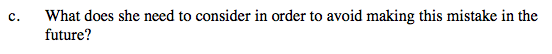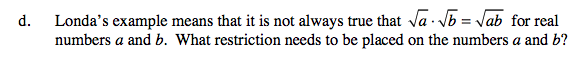### Home > A2C > Chapter Ch9 > Lesson 9.3.2 > Problem9-163

9-163.First eliminate the negative sign from each radical by replacing it with i.

Remember that i is the square root of −1, now finish multiplying.

−7$\text{Londa multiplied }\sqrt{-7}\cdot\sqrt{-7}\text{ to get }\sqrt{49} = 7$See parts (a) and (b).The variables a and b must be non-negative real numbers.